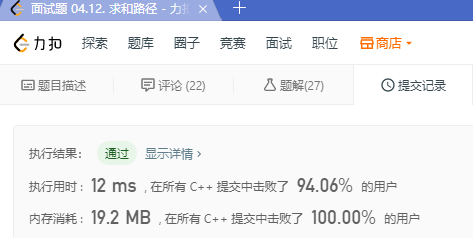# 程序员面试金典 - 面试题 04.12. 求和路径（二叉树递归）

## 1. 题目

示例:

5
/ \
4   8
/   / \
11  13  4
/  \    / \
7    2  5   1

3



## 2. 解题

• 遍历每个节点，并从该节点开始计算和
class Solution {
int count = 0;
public:
int pathSum(TreeNode* root, int sum) {
if(!root) return 0;
calsum(root,0,sum);
pathSum(root->left,sum);
pathSum(root->right,sum);
return count;
}

void calsum(TreeNode* root, int s, int sum)
{
if(!root)
return;
if(s+root->val == sum)
count++;
calsum(root->left,s+root->val,sum);
calsum(root->right,s+root->val,sum);
}
};©️2019 CSDN 皮肤主题: 技术黑板 设计师: CSDN官方博客